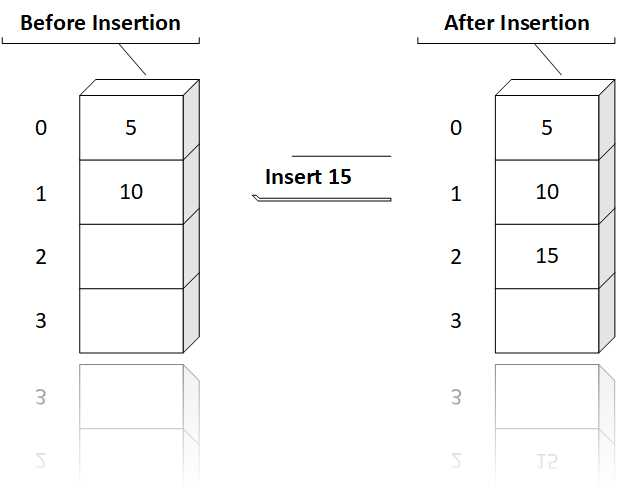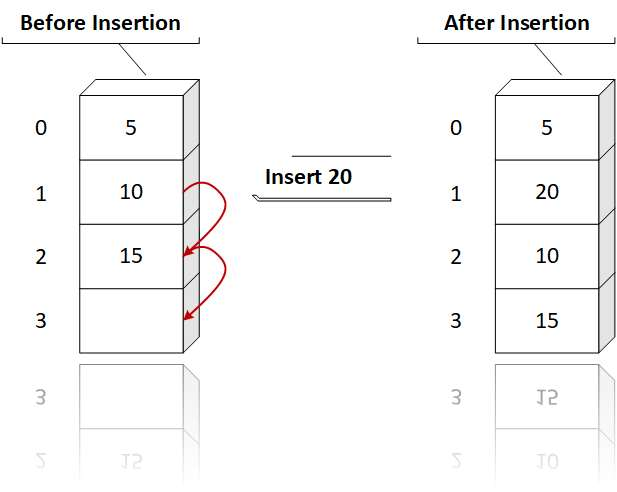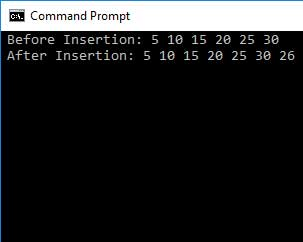# Array C Programing

Answer: Insertion refers to adding an element to existing list of elements. After insertion the size of the linear array is increased by a factor of one. Insertion is possible only if the memory space allocated is large enough to accomodate the additional element. Inserting at the end of linear list can be done very easily.

However, to insert an element at any other location, the elements are to be moved downward to new locations to accomodate the new element and keep the order of other elements.

Insertion can be done either at the end of array or at a given position.

Insertion at End:Insertion at the end of array is very simple, we have to just find the last element of array and insert the element after that location.

Insertion at a Given Position :Insertion at a given position is a time consuming and expensive process, as it results in shifting the array elements, to make room for a new element.

Code:

``````
// C program to implement insert
// operation in an unsorted array.
#include

// Inserts a key in arr[] of given capacity.
// n is current size of arr[]. This
// function returns n + 1 if insertion
// is successful, else n.
int insertSorted(int arr[], int n,
int key,
int capacity)
{

// Cannot insert more elements if n is
// already more than or equal to capcity
if (n >= capacity)
return n;

arr[n] = key;

return (n + 1);
}

// Driver Code
int main()
{
int arr = {5, 10, 15, 20, 25, 30};
int capacity = sizeof(arr) / sizeof(arr);
int n = 6;
int i, key = 26;

printf("\n Before Insertion: ");
for (i = 0; i < n; i++)
printf("%d ", arr[i]);

// Inserting key
n = insertSorted(arr, n, key, capacity);

printf("\n After Insertion: ");
for (i = 0; i < n; i++)
printf("%d ",arr[i]);

return 0;
}

``````

Output: